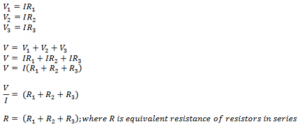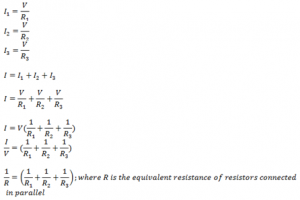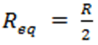## TyroCity

Physics XII Notes for Physics Notes

Posted on • Updated on

# Combination Of Resistors

Series combination
If resistors are connected end to end such that same current flows in each resistor but different potential difference is observed across then such combination is called series combination.

Let, R1, R2 and R3be the three resistors connected in series to a battery of volt V. Same current I flows in each resistors while V1, V2 and V3potential drops are observed across R1, R2 and R3 respectively.Parallel combination
If one of the ends of resistors are connected to a common point and the rest of the ends to another common point such that same potential difference is observed across each resistor but different current in each resistor than such combination is called parallel combination.

Let, R1, R2 and R3be the three resistors connected in parallel to a battery of volt V. Current I1, I2 and I3 flows across resistance R1, R2and R3 while potential drops V is observed across each resistor. Then,The equivalent resistance is smaller than the smallest individual resistors connected in parallel.

Some rules

• If identical resistors each of resistance R are connected in series than the equivalent resistance is “Req = nR” so we get maximum resistance from the given resistors when we connect them in series.
• If two identical resistors each of resistance R are connected in parallel then,• The ratio of maximum resistance to the minimum resistance obtained by connecting n identical resistors in series and in parallel is n2.